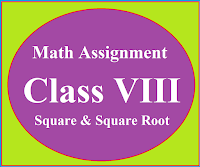### Math Assignment Class VIII | Square & Square RootMath Assignment  Class VIII | Square & Square Root Download or Print free  assignment with answer key  for   Class  8 Squares and  Square Roots.   Important and extra questions that cover all topics of square and square root and is useful and helpful for the students. Math Assignment  Class VIII | Square & Square Root LEVEL -1

### Class XII Maths Formulas | Basics | Assignments

 Chapter-wise Basics and Formulas

CBSE syllabus, sample papers, unit tests and solutions of sample papers, CBSE syllabus for class XII for all subjects, chapter-wise basic concepts of mathematics for class XII, chapter-wise planning in mathematics for class XII.

*******************************************

## TEACHERS NOTESSUBJECT: MATHEMATICS CLASS XII

 CLASS 12 (Non- Medical and Applied Mathematics)
***************************************

## MATHS FORMULASMATHS ASSIGNMENTSMATHS (MCQ)BASIC POINTS IN DETAIL

CHAPTER 1: Relations and Functions

Basic & Formulas

Multiple Choice Questions Ch-1

CHAPTER 2: (Inverse Trigonometric Functions)

Basics & Formulas

Assignment 1

Multiple Choice Questions Ch-2

CHAPTER 3: (Matrices)

Basics & Formula's

Sol. of Important Questions Ch-3

Assignment Ch-3

Multiple Choice Questions Ch-3

CHAPTER 4: Determinants

Basics & Formulas

Assignment Ch-04

Multiple Choice Questions Ch-4
CHAPTER 5: Continuity and Differentiability

Basics & Formulas

Limit & Continuity

Assignment 1

Multiple Choice Questions Ch-5

CHAPTER 6: Application of Derivatives

Basics & Formulas

Multiple Choice Questions Ch-6

 CHAPTER 7: Integral (Indefinite and Definite)Indefinite IntegralDefinite IntegralMultiple Choice Questions Ch-7 CHAPTER 8: Application of IntegralsBasic & Formula'sConic Section - 1 (Circle and Parabola)Conic Section - 2 (Ellipse and Hyperbola)Multiple Choice Questions Ch-8 CHAPTER 9: Differential EquationsMultiple Choice Questions Ch-9 CHAPTER 10: Vector AlgebraBasics & Formulas CHAPTER 11: Three Dimensional GeometryBasics & FormulasAssignmentMultiple Choice Questions Ch-11 CHAPTER 12: Linear Programming problems (LPP)Basics & Formula'sMultiple Choice Questions  Ch-12 CHAPTER 13: ProbabilityProbability - 1Probability - 2Multiple Choice Questions Ch-13

 STUDY MATERIAL CLASS 12 CBSE  Syllabus (Mathematics) For  (Classes 11 & 12)  2022-23 : Click here Deleted portion in mathematics for 2022-23 : Click Here

## Common errors made by the students while solving the questions

 NCERT Mathematics Book with solutions NCERT Book NCERT Sol. NCERT Book NCERT Sol.
****************************************